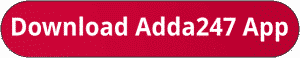Latest Banking jobs   »   sbi quant

# Quantitative Aptitude Quiz For SBI Clerk Prelims 2023-13th October

Q1. Akshay buys an article and markup it 30 % above its cost price. At the time of sale, he gives 10% discount instead of 15% due to which he earns Rs. 13 more. Find cost price.
(a) Rs. 230
(b) None of these
(c) Rs. 150
(d) Rs. 130
(e) Rs. 200Q3. Rs. 12000 becomes Rs. 15000 in 18 months at a certain rate of interest at simple interest. Find amount if Rs. 5000 invested at same rate for 30 months at simple interest.
(a) Rs. 7883.33
(b) Rs. 7083.33
(c) Rs. 7279.80
(d) Rs. 7173.33
(e) None of these

Q4. Johny calculates his profit at cost price while Jini at selling price. If cost price is same for all and everyone calculate their profit as 10%. Find ratio of selling price.
(a) 100:111
(b) 10:11
(c) 10:101
(d) 99:100
(e) Cannot be determined

Q5. A sum when invested for a year at 20% compounding annually would fetch Rs. 10 less when compounding is done half-yearly. Find the sum invested.
(a) Rs. 850
(b) Rs. 950
(c) Rs. 1000
(d) Rs. 1100
(e) Rs. 1050

Q6.When an article was sold at 3/5 th of the marked price, the loss percentage was 10%. The article should be sold at what fraction of marked price to earn 10% profit?
(a) 3/7
(b) 11/15
(c) 7/9
(d) 13/19
(e) 4/5

Q7. A shopkeeper marked up the price of a bag by 20% while gave two discounts of 10% and d%. If he has allowed only first discount then he would have gained Rs 27 more. Find CP if he gained Rs 13 in whole transaction.
(a) Cannot be determined
(b) Rs 540
(c) Rs 600
(d) Rs 500
(e) None of these

Q8. Chandu invested Rs 1500 in a scheme offering 10% SI. After 2 years he invested a part of amount he received again in the same scheme for 3 years from which he received Rs 300. Find the amount he did not invest again.
(a) Rs. 850
(b) Rs. 1000
(c) Rs. 950
(d) Rs. 800
(e) Rs. 1050

Q9. A man invested Rs.P in three different schemes – A, B and C in the ratio 2 : 1 : 3 respectively. He invested in scheme A at the rate of 10% p.a. at SI for 2 years, in scheme B at the rate of 5% p.a at C.I compounded annually for 2 years and in scheme C at the rate of 6% p.a at CI compounded half yearly for 1 year and received total interest of Rs. 6852. Find the value of P.
(a) Rs.60000
(b) Rs.72000
(c) Rs.48000
(d) Rs.120000
(e) can’t be determined.

Q10. A and B started a business with some amount. After 9 months B left the business & C joins the business with Rs. 12,000 and remains in business till the end of year. At the end of the year, profit share of A, B and C is Rs. 48, Rs. 48 and Rs. 24 respectively. Find the sum of the amount (in Rs.) invested by A and B together in the business?
(a) 8,000
(b) 10,000
(c) 15,000
(d) 12,000
(e) 14,000

Solutions. .               .                                      .       .

## FAQs

### What is the selection process of SBI Clerk 2023?

The selection process of SBI Clerk 2023 consists of Prelims, Mains and LPT.

#### Congratulations!Union Budget 2023-24: Free PDF